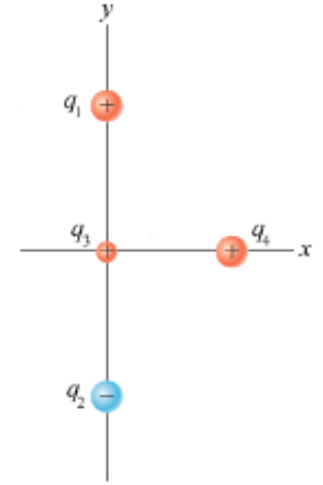# Problem: Two particles, with charges of q1 = 50.0 nC and q2 = -50.0 nC, are placed at the points with coordinates (0, 12.00 cm) and (0, -12.00 cm) as shown in the figure below. A particle with charge q3 = 25.00 nC is located at the origin.(a) Find the electric potential energy of the configuration of the three fixed charges.(b) A fourth particle, with a mass of 2.01x10-13 kg and a charge of q4 = 100.0 nC, is released from rest at the point (9.00 cm, 0). Find its speed after it has moved freely to a very large distance away.

###### FREE Expert Solution

The electric potential energy is given by:

$\overline{){\mathbf{U}}{\mathbf{=}}\frac{\mathbf{k}{\mathbf{q}}_{\mathbf{1}}{\mathbf{q}}_{\mathbf{2}}}{{\mathbf{r}}_{\mathbf{12}}}}$

We get the speed of the particle from the conversation of energy:

$\overline{)\begin{array}{rcl}{\mathbf{K}}& {\mathbf{=}}& {\mathbf{U}}\\ \frac{\mathbf{1}}{\mathbf{2}}\mathbf{m}{\mathbf{v}}^{\mathbf{2}}& {\mathbf{=}}& \mathbf{k}\frac{{\mathbf{q}}_{\mathbf{3}}{\mathbf{q}}_{\mathbf{4}}}{{\mathbf{r}}_{\mathbf{34}}}\end{array}}$

97% (468 ratings)###### Problem DetailsTwo particles, with charges of q1 = 50.0 nC and q2 = -50.0 nC, are placed at the points with coordinates (0, 12.00 cm) and (0, -12.00 cm) as shown in the figure below. A particle with charge q3 = 25.00 nC is located at the origin.

(a) Find the electric potential energy of the configuration of the three fixed charges.

(b) A fourth particle, with a mass of 2.01x10-13 kg and a charge of q4 = 100.0 nC, is released from rest at the point (9.00 cm, 0). Find its speed after it has moved freely to a very large distance away.